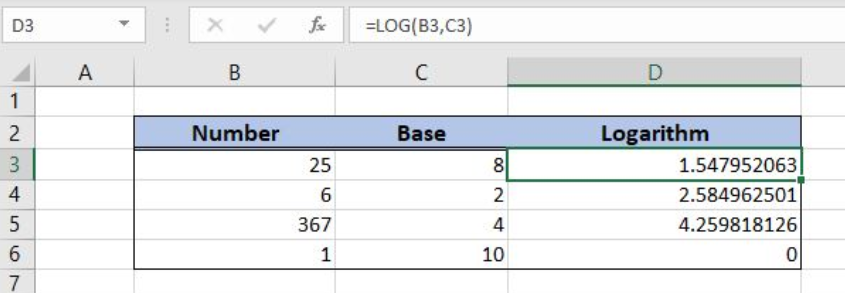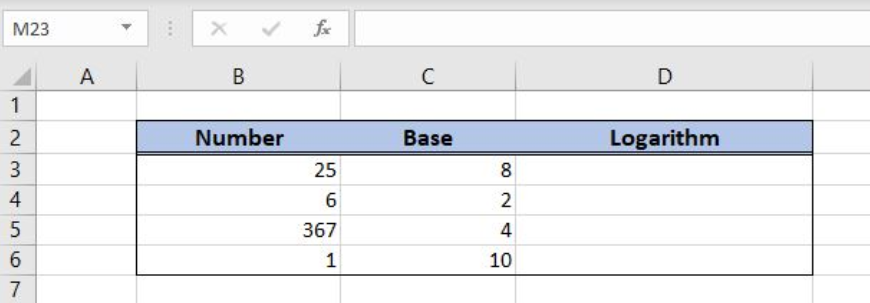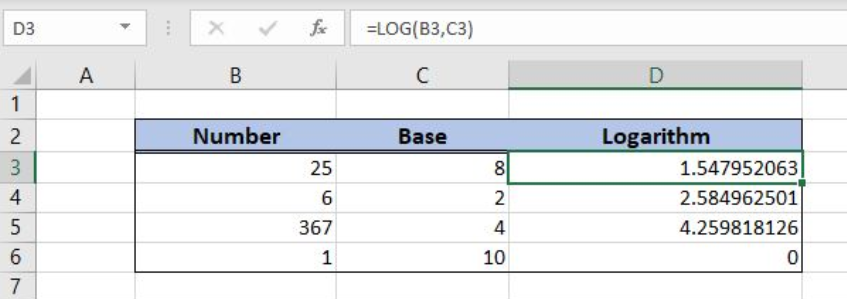Get instant live expert help with Excel or Google Sheets“My Excelchat expert helped me in less than 20 minutes, saving me what would have been 5 hours of work!”

#### Post your problem and you'll get expert help in seconds

Your message must be at least 40 characters
Our professional experts are available now. Your privacy is guaranteed.

# Excel LOG Function

Excel allows us to calculate the logarithm of a number for a given base, by using the LOG function. This step by step tutorial will assist all levels of Excel users in getting the logarithm for a numberFigure 1. The result of the LOG function

## Syntax of the LOG Formula

The generic formula for the LOG function is:

`=LOG(number, base)`

The parameters of the LOG function are:

• number – a number for which we want to calculate the logarithm
• base – a base for the logarithm calculation. This parameter is optional. If we omit it, the function will take 10 as a base.

## Setting up Our Data for the LOG Function

In column B (“Number”), we have the list of numbers and in column C (“Base”), a base for each number. In column D (“Logarithm”), we want to get the logarithm for number and base.Figure 2. Data that we will use in the LOG example

## Calculate the Logarithm Using the LOG Function

In our example, we want to get the logarithm in the cell D3, for 25 and base 8.

The formula in the cell D3 is:

`=LOG(B3, C3)`

The parameter number is the cell B3, while the parameter base is C3.

To apply the LOG function, we need to follow these steps:

• Select cell C3 and click on it
• Insert the formula: `=LOG(B3)`
• Press enter
• Drag the formula down to the other cells in the column by clicking and dragging the little “+” icon at the bottom-right of the cell.Figure 3. Using the LOG function

As you can see in the cell D3, the logarithm for 25 and base 8 is 1.547952063.

## Notes

When using the LOG  function, these are the possible errors:

• #VALUE! Error occurs if a number or a base are non-numeric
• #NUM! Error occurs if a number or a base are negative or zero
• #DIV/0! Error occurs if a base is 1.

Most of the time, the problem you will need to solve will be more complex than a simple application of a formula or function. If you want to save hours of research and frustration, try our live Excelchat service! Our Excel Experts are available 24/7 to answer any Excel question you may have. We guarantee a connection within 30 seconds and a customized solution within 20 minutes.